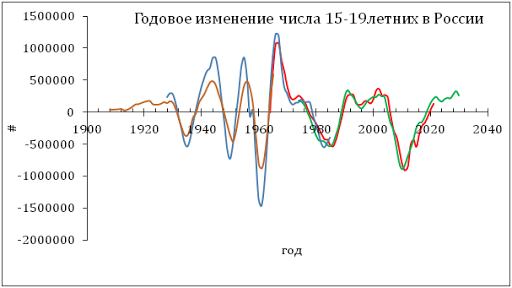## 1/27/12

### Real GDP and GDP deflator in 2011

Here are some quick notes on the new estimates of real GDP and GDP deflator for 2011.  Figurer 1 shows the rate of growth of real GDP, dlnGDP/dt, at annual and quarterly basis. In 2011, the rate is 0.017 1/y, i.e. 1.7% per year, despite the rate growth in the fourth quarter of 2.7% (SAAR). Previously, we predicted a small recession in 2012 and 2013. This prediction will be updated soon when the 2010 census results are published and incorporated into the so called postcensal population estimates.
Figure 2 shows the growth of total population for the purpose of per head calculations. Please notice a large step in population between 1999 and 2000 as associated with the error of closure, i.e. the difference between intercensal estimate for 2000 and the number enumerated in the 2000 census.  Figure 3 presents the rate of growth of real GDP per capita , dlnG/dt. In 2011, the rate of growth was 0.0098 1/y. This means that the total increase in population was of 0.7%.
Figure 4 shows the GDP deflator or price inflation associated with the economy as a whole. This is the most comprehensive measure of inflation as we discussed many times in this blog. For 2011, the GDP deflator is 2.1%. This is larger than we predicted but the last quarter signals about upcoming deflation, as we foresaw six years ago. Currently, the FRB also foresees very low inflation rates through 2014.
According to our concept of GDP growth,  real GDP per capita has a inertial component which is expressed in a constant annual increment, dG=const. Figure 5 updates the graph showing the evolution of real GDP per capita in the USA. One can observe a gradual return to the constant level of annual increment. This works as inertial movement in physics. However, one can expect some more years of dG less than average, dG<\$490 (2011 US dollars).  It is worth noting, that there is no output gap when real GDP per cpaita is considered. The evolution of dG exactly follows it long term trend and the years after 2007 serve to return dG to the trend from its highs in the late 1990s.
We have to notice that the estimates of the nominal GDP and GDP deflator are subject to revision which may be as high as several per cent (+2.1% for 2001). However, the long term trends in all presented variables fit our concept and predictions.

Figure 1. The growth rate of real GDP: annual and quarterly (annualized). MA(4) for the quarterly time series. For 2011, dlnGDP/dt=0.017  1/y.

Figure 2. The evolution of total resident population. Notice the jump between 1999 and 2000 – the closure error.

Figure 3. The growth rate of real GDP per capita. For 2011, the rate is (dlnG/dt=) 0.0098 1/y.
Figure 4. Annual and quarterly (annualized) price deflator of GDP. In the last quarter of 2011 the GDP deflator dropped to 0.004 1/y. This is likely a turn to deflation.

Figure 5. The increment of real GDP per capita since 1950. As predicted, the trend returns to a zero slope. There is no output gap.